# KR20140124448A - Method for encoding and decoding video using intra prediction, and apparatus thereof - Google Patents

Method for encoding and decoding video using intra prediction, and apparatus thereof Download PDF

## Info

Publication number
KR20140124448A
KR20140124448A KR1020130041304A KR20130041304A KR20140124448A KR 20140124448 A KR20140124448 A KR 20140124448A KR 1020130041304 A KR1020130041304 A KR 1020130041304A KR 20130041304 A KR20130041304 A KR 20130041304A KR 20140124448 A KR20140124448 A KR 20140124448A
Authority
KR
South Korea
Prior art keywords
prediction
mode
intra
base
difference
Prior art date
Application number
KR1020130041304A
Other languages
Korean (ko)
Inventor
문주희
최광현
한종기
Original Assignee
인텔렉추얼디스커버리 주식회사
Priority date (The priority date is an assumption and is not a legal conclusion. Google has not performed a legal analysis and makes no representation as to the accuracy of the date listed.)
Filing date
Publication date
Application filed by 인텔렉추얼디스커버리 주식회사 filed Critical 인텔렉추얼디스커버리 주식회사
Priority to KR1020130041304A priority Critical patent/KR20140124448A/en
Priority claimed from US14/784,467 external-priority patent/US20160073107A1/en
Publication of KR20140124448A publication Critical patent/KR20140124448A/en

• 206010057190 Respiratory tract infection Diseases 0.000 claims description 2
• 238000010586 diagram Methods 0.000 description 15
• 238000000034 method Methods 0.000 description 15
• 230000003044 adaptive Effects 0.000 description 9
• 238000001914 filtration Methods 0.000 description 6
• 241001442055 Vipera berus Species 0.000 description 4
• 238000006243 chemical reaction Methods 0.000 description 4
• 239000011159 matrix material Substances 0.000 description 4
• 230000001131 transforming Effects 0.000 description 4
• 230000005540 biological transmission Effects 0.000 description 2
• 238000007906 compression Methods 0.000 description 2
• 238000007796 conventional method Methods 0.000 description 2
• 230000000875 corresponding Effects 0.000 description 2
• 230000000903 blocking Effects 0.000 description 1
• 239000000969 carrier Substances 0.000 description 1
• 239000000470 constituent Substances 0.000 description 1
• 230000003247 decreasing Effects 0.000 description 1
• 230000000694 effects Effects 0.000 description 1
• 238000009499 grossing Methods 0.000 description 1
• 238000004519 manufacturing process Methods 0.000 description 1
• 239000000463 material Substances 0.000 description 1
• 230000004048 modification Effects 0.000 description 1
• 238000006011 modification reaction Methods 0.000 description 1
• 230000003287 optical Effects 0.000 description 1
• 230000002093 peripheral Effects 0.000 description 1

## Images

•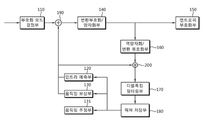••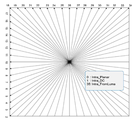•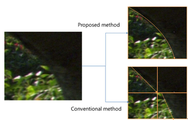•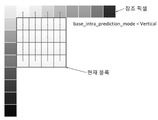•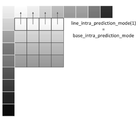•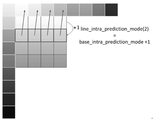•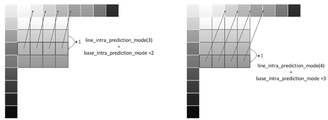•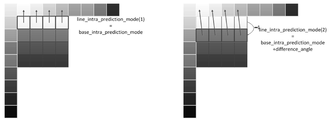•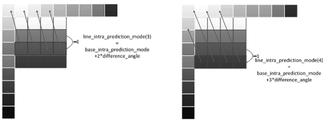•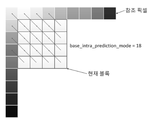•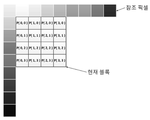•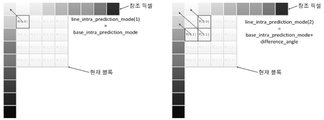•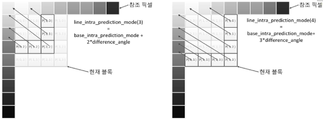•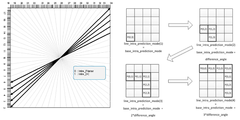•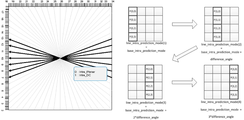•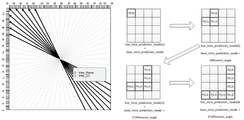•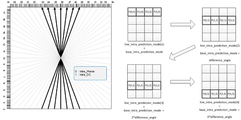•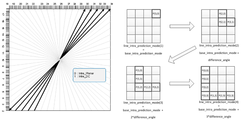•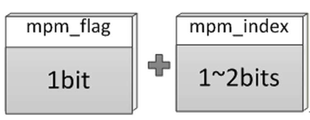•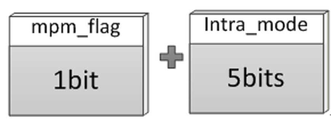•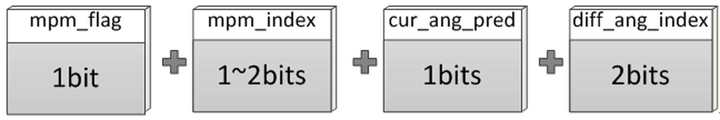•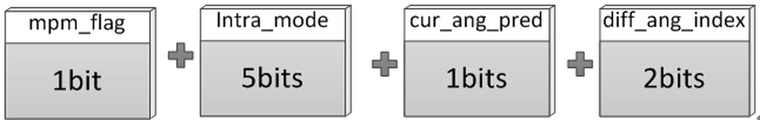•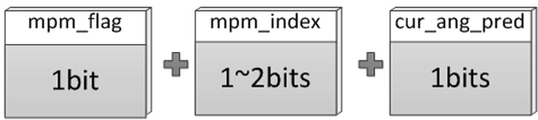•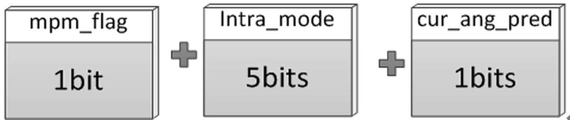•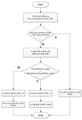•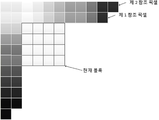•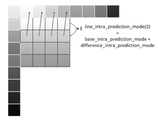•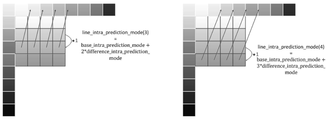•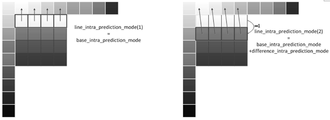•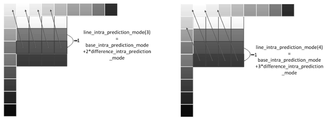•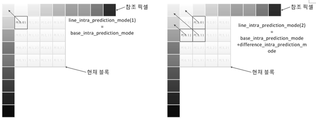•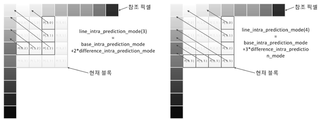•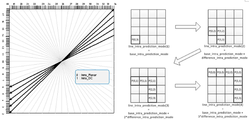•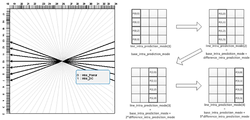•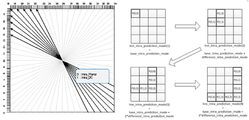•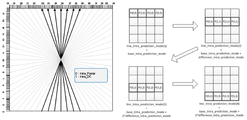•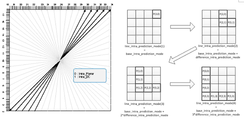•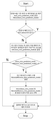•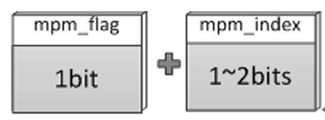•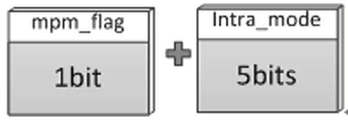•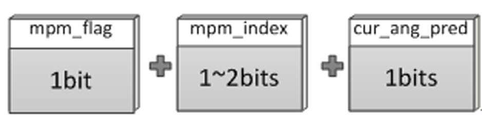•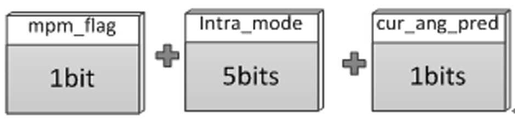•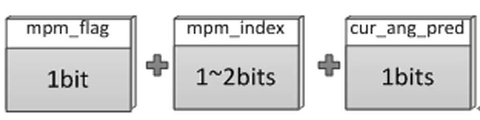•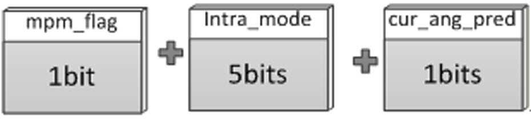•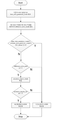## Classifications

• HELECTRICITY
• H04ELECTRIC COMMUNICATION TECHNIQUE
• H04NPICTORIAL COMMUNICATION, e.g. TELEVISION
• H04N19/00Methods or arrangements for coding, decoding, compressing or decompressing digital video signals
• H04N19/10Methods or arrangements for coding, decoding, compressing or decompressing digital video signals using adaptive coding
• H04N19/102Methods or arrangements for coding, decoding, compressing or decompressing digital video signals using adaptive coding characterised by the element, parameter or selection affected or controlled by the adaptive coding
• H04N19/103Selection of coding mode or of prediction mode
• HELECTRICITY
• H04ELECTRIC COMMUNICATION TECHNIQUE
• H04NPICTORIAL COMMUNICATION, e.g. TELEVISION
• H04N19/00Methods or arrangements for coding, decoding, compressing or decompressing digital video signals
• H04N19/10Methods or arrangements for coding, decoding, compressing or decompressing digital video signals using adaptive coding
• H04N19/134Methods or arrangements for coding, decoding, compressing or decompressing digital video signals using adaptive coding characterised by the element, parameter or criterion affecting or controlling the adaptive coding
• H04N19/136Incoming video signal characteristics or properties
• HELECTRICITY
• H04ELECTRIC COMMUNICATION TECHNIQUE
• H04NPICTORIAL COMMUNICATION, e.g. TELEVISION
• H04N19/00Methods or arrangements for coding, decoding, compressing or decompressing digital video signals
• H04N19/10Methods or arrangements for coding, decoding, compressing or decompressing digital video signals using adaptive coding
• H04N19/169Methods or arrangements for coding, decoding, compressing or decompressing digital video signals using adaptive coding characterised by the coding unit, i.e. the structural portion or semantic portion of the video signal being the object or the subject of the adaptive coding
• H04N19/17Methods or arrangements for coding, decoding, compressing or decompressing digital video signals using adaptive coding characterised by the coding unit, i.e. the structural portion or semantic portion of the video signal being the object or the subject of the adaptive coding the unit being an image region, e.g. an object
• H04N19/176Methods or arrangements for coding, decoding, compressing or decompressing digital video signals using adaptive coding characterised by the coding unit, i.e. the structural portion or semantic portion of the video signal being the object or the subject of the adaptive coding the unit being an image region, e.g. an object the region being a block, e.g. a macroblock
• HELECTRICITY
• H04ELECTRIC COMMUNICATION TECHNIQUE
• H04NPICTORIAL COMMUNICATION, e.g. TELEVISION
• H04N19/00Methods or arrangements for coding, decoding, compressing or decompressing digital video signals
• H04N19/50Methods or arrangements for coding, decoding, compressing or decompressing digital video signals using predictive coding
• H04N19/593Methods or arrangements for coding, decoding, compressing or decompressing digital video signals using predictive coding involving spatial prediction techniques

## Abstract

According to an embodiment of the present invention, there is provided a method of encoding a video, the method comprising: determining a coding mode for a video block; Determining whether a curved prediction mode is to be performed if the coding mode is intra prediction; And performing intra coding according to the curved prediction and the base intra prediction mode.

## Description

BACKGROUND OF THE INVENTION 1. Field of the Invention The present invention relates to a video encoding / decoding method and apparatus using intra prediction,
The present invention relates to a video codec, and more particularly, to a method and apparatus for providing a video codec using a curved prediction technique.
In general HEVC Intra Prediction process, Intra prediction is performed in 34 directions of linear shape. This method has a limitation in encoding efficiency in a portion having a curved edge or a region in which the brightness gradually changes (such as a background sky portion in a Kimono sequence). In this case, contour (contour line) error occurs.
Embodiments of the present invention provide a method and apparatus for overcoming coding error limit and contour error in intra prediction in a video codec.
It is to be understood, however, that the technical scope of the present invention is not limited to the above-described technical problems, and other technical problems may be present.
According to a first aspect of the present invention, there is provided a method of providing a video codec, the method comprising the steps of: performing curve prediction in intraprediction; An interpolation method, an MPM determination method, a transform method, and a scanning method.
According to an embodiment of the present invention, there is provided a method of encoding a video, the method comprising: determining a coding mode for a video block; Determining whether a curved prediction mode is to be performed if the coding mode is intra prediction; And performing intra coding according to the curved prediction and the base intra prediction mode.
According to the embodiment of the present invention, the coding efficiency can be improved by performing curved prediction in Intra Prediction or a portion having a curved edge or a region in which luminance gradually changes (such as a background sky portion in a Kimono sequence).
In the future, the HEVC will compress the image signal with a much higher resolution than the 4K image that the HEVC is aiming to encode, and the effect of this patented technology in this environment will appear very efficiently.
1 is a block diagram showing an example of a configuration of a video encoding apparatus.
2 is a block diagram showing an example of a structure of a video decoding apparatus.
3 is a diagram showing an example of intra prediction modes.
4 is a diagram for explaining a method of determining an intra-prediction direction pattern according to an embodiment of the present invention.
5 is a diagram illustrating an embodiment of a method for determining an intra-prediction direction pattern according to a mode occurrence frequency.
6 is a diagram illustrating an embodiment of a method for determining an intra-prediction direction pattern according to edge information.
Hereinafter, embodiments of the present invention will be described in detail with reference to the accompanying drawings so that those skilled in the art can easily carry out the present invention. It should be understood, however, that the present invention may be embodied in many different forms and should not be construed as limited to the embodiments set forth herein. In the drawings, the same reference numbers are used throughout the specification to refer to the same or like parts.
Throughout this specification, when a part is referred to as being "connected" to another part, it is not limited to a case where it is "directly connected" but also includes the case where it is "electrically connected" do.
Throughout this specification, when a member is located on another member, this includes not only when a member is in contact with another member but also when another member is present between the two members.
Throughout this specification, when an element is referred to as "including " an element, it is understood that the element may include other elements as well, without departing from the other elements unless specifically stated otherwise. The terms "about "," substantially ", etc. used to the extent that they are used throughout the specification are intended to be taken to mean the approximation of the manufacturing and material tolerances inherent in the stated sense, Accurate or absolute numbers are used to help prevent unauthorized exploitation by unauthorized intruders of the referenced disclosure. The word " step (or step) "or" step "used to the extent that it is used throughout the specification does not mean" step for.
Throughout the present specification, the term of a combination of these in the expression of a mark-up form means a combination or combination of at least one selected from the group consisting of the constituents described in the expression of the mark-up form, Quot; is meant to include one or more selected from the group consisting of
As an example of a method of encoding an actual image and its depth information map, the Moving Picture Experts Group (MPEG) and the Video Coding Experts Group (VCEG) having the highest coding efficiency among the video coding standards developed so far jointly standardize Encoding can be performed using HEVC (High Efficiency Video Coding).
In one embodiment of the present invention, curve prediction is performed at Intra Prediction.
The video codec technology according to an exemplary embodiment of the present invention proposes prediction filters by curve type and mode.
The video codec technology according to an embodiment of the present invention proposes a filtering method of neighboring pixels (similar to Intra Smoothing of current HEVC) according to a curve prediction mode and a mode.
The video codec technology according to an embodiment of the present invention proposes a method of interpolating neighboring pixels to apply a curved prediction filter.
The video codec technology according to an exemplary embodiment of the present invention proposes a method of encoding curve shape and mode information. At this time, MPM decision method is proposed according to the curve prediction mode and mode.
The video codec technology according to an exemplary embodiment of the present invention uses different transforms according to curve shape and mode information.
The video codec technology according to an exemplary embodiment of the present invention uses different transform coefficient scanning methods according to curve shape and mode information.
FIG. 1 is a block diagram illustrating an example of a configuration of a video encoding apparatus. The encoding apparatus includes a coding mode determination unit 110, an intra prediction unit 120, a motion compensation unit 130, a motion estimation unit 131 Quantization unit 140, an entropy encoding unit 150, an inverse quantization / transformation decoding unit 160, a deblocking filtering unit 170, a picture storage unit 180, a subtraction unit 190, (200).
Referring to FIG. 1, an encoding mode determination unit 110 analyzes an input video signal to divide a picture into a predetermined size of an encoding block, and determines a coding mode for a predetermined size of the encoding block. The encoding mode includes intraprediction encoding and inter prediction encoding.
The picture is composed of a plurality of slices, and the slice is composed of a plurality of maximum coding units (LCU). The LCU can be divided into a plurality of coding units (CUs), and the encoder can add information indicating whether or not to be divided to a bit stream. The decoder can recognize the position of the LCU by using the address (LcuAddr). The coding unit CU in the case where division is not allowed is regarded as a prediction unit (PU), and the decoder can recognize the position of the PU using the PU index.
The prediction unit PU may be divided into a plurality of partitions. Also, the prediction unit PU may be composed of a plurality of conversion units (TUs).
The encoding mode determination unit 110 sends the image data to the subtraction unit 190 in units of blocks of a predetermined size (for example, in units of PU or TU) according to the determined encoding mode.
The transform coding / quantizing unit 140 transforms the residual block calculated by the subtracting unit 190 from the spatial domain to the frequency domain.
For example, two-dimensional discrete cosine transform (DCT) or discrete cosine transform (DST) -based transform is performed on the residual block. In addition, the transcoding / quantization unit 140 determines a quantization step size for quantizing the transform coefficient, and quantizes the transform coefficient using the determined quantization step size. The quantization matrix can be determined according to the determined quantization step size and encoding mode.
The quantized two-dimensional transform coefficients are transformed into one-dimensional quantized transform coefficients by one of the predetermined scanning methods.
The transformed one-dimensional sequence of quantization transform coefficients is supplied to the entropy encoding unit 150.
The inverse quantization / conversion decoding unit 160 dequantizes the quantization coefficients quantized by the transcoding / quantization unit 140. Also,
The inverse quantization coefficient obtained by inverse quantization is inversely transformed. Accordingly, the residual block transformed into the frequency domain can be restored into the residual block in the spatial domain.
The deblocking filtering unit 170 receives the inverse quantized and inverse transformed image data from the inverse quantization / inverse transform coding unit 160 and performs filtering to remove a blocking effect.
The picture storage unit 180 receives the filtered image data from the deblocking filtering unit 170 and restores and restores the image in picture units. The picture may be a frame-based image or a field-based image.
The picture storage unit 180 has a buffer (not shown) capable of storing a plurality of pictures. A plurality of pictures stored in the buffer are provided for intra prediction and motion estimation. The pictures provided for intra prediction or motion estimation are referred to as reference pictures.
The motion estimation unit 131 receives the at least one reference picture stored in the picture storage unit 180 and performs motion estimation to output motion data including an index indicating a motion vector and a reference picture and a block mode do.
In order to optimize the prediction precision, a motion vector is determined with a fractional pixel precision, for example, 1/2 or 1/4 pixel accuracy. Since the motion vector can have a fractional pixel precision, the motion compensation unit 130 applies the interpolation filter for calculating the pixel value of the fractional pixel position to the reference picture so that the pixel value of the fractional pixel position .
The motion compensation unit 130 is configured to perform motion compensation on a block to be coded from a reference picture used for motion estimation among a plurality of reference pictures stored in the picture storage unit 180 according to the motion data input from the motion estimation unit 131 And outputs the extracted prediction block.
The motion compensation unit 130 determines a filter characteristic of the adaptive interpolation filter necessary for motion compensation with a decimal precision. The filter characteristic is, for example, information indicating the filter type of the adaptive interpolation filter and information indicating the size of the adaptive interpolation filter. The size of the filter is, for example, the number of taps, which is the number of filter coefficients of the adaptive interpolation filter.
Specifically, the motion compensation unit 130 may determine either a separate type or a non-separable type adaptive filter as an adaptive interpolation filter. Then, the number of taps of the determined adaptive interpolation filter and the value of each filter coefficient are determined. The value of the filter coefficient can be determined differently for each position of the fractional pixel relative to the integer pixel. Also, the motion compensation unit 130 may use a plurality of non-adaptive interpolation filters with fixed filter coefficients.
The motion compensation unit 130 can set the characteristics of the interpolation filter in a predetermined processing unit. For example, it can be set in a fractional pixel unit, a coding basic unit (encoding unit), a slice unit, a picture unit, or a sequence unit. In addition, one characteristic may be set for one video data. Therefore, since the same filter characteristic is used in a predetermined processing unit, the motion compensation unit 130 has a memory that temporarily holds the filter characteristic. This memory maintains filter characteristics, filter coefficients, and the like as needed. For example, the motion compensation unit 130 can determine the filter characteristic for each I picture and determine the filter coefficient for each slice.
The motion compensation unit 130 receives a reference picture from the picture storage unit 180 and applies a filter process using the determined adaptive interpolation filter to generate a prediction reference picture of a decimal precision.
Then, based on the generated reference picture and the motion vector determined by the motion estimation unit 131, motion compensation is performed with a small number of pixels to generate a prediction block.
The subtractor 190 receives the block in the reference picture corresponding to the input block from the motion compensator 130 and performs a difference operation with the input macroblock in the case of performing inter picture prediction coding on the input block to be coded, and outputs a residue signal.
The intraprediction unit 120 performs intraprediction encoding using the reconstructed pixel values in a picture to be predicted. The intra prediction unit receives the current block to be predictively encoded and performs intra prediction by selecting one of a plurality of intra prediction modes preset according to the size of the current block. The intra predictor 120 determines the intra prediction mode of the current block using the previously coded pixels adjacent to the current block, and generates a prediction block corresponding to the determined mode.
The previously encoded region of the current picture is decoded again for use by the intra prediction unit 120 and stored in the picture storage unit 180. [ The intra prediction unit 120 generates a prediction block of a current block using pixels neighboring the current block or non-adjacent but applicable pixels in the previously coded area of the current picture stored in the picture storage unit 180. [
The intra prediction unit 120 may adaptively filter adjacent pixels to predict an intra block. For the same operation in the decoder, it is possible to transmit information indicating whether or not filtering is performed in the encoder. Or the intra-prediction mode of the current block and the size information of the current block.
The prediction type used by the image coding apparatus depends on whether the input block is coded in the intra mode or the inter mode by the coding mode determination unit.
The switching between the intra mode and the inter mode is controlled by the intra / inter selector switch.
The entropy encoding unit 150 entropy-codes the quantization coefficients quantized by the transcoding / quantization unit 140 and the motion information generated by the motion estimation unit 131. [ Also, an intra prediction mode, control data (e.g., quantization step size, etc.), and the like can be coded. Also, the filter coefficient determined by the motion compensation unit 130 is encoded and output as a bit stream.
FIG. 2 is a block diagram illustrating an example of a configuration of a video decoding apparatus. The illustrated decoding apparatus includes an intropy decoding unit 210, an inverse quantization / inverse transform unit 220, an adder 270, a deblocking filter unit 250, a picture storage unit 260, an intra prediction unit 230, a motion compensation prediction unit 240, and an intra / inter changeover switch 280.
Referring to FIG. 2, the intropy decoding unit 210 decodes an encoded bitstream transmitted from a moving picture encoding apparatus into an intra-prediction mode index, motion information, and a quantized coefficient sequence. The intropy decoding unit 210 supplies the decoded motion information to the motion compensation prediction unit 240. [ The intropy decoding unit 210 supplies the intra prediction mode index to the intraprediction unit 230 and the inverse quantization / inverse transformation unit 220. Also, the intropy decoding unit 210 supplies the inverse quantization coefficient sequence to the inverse quantization / inverse transformation unit 220.
The inverse quantization / inverse transform unit 220 transforms the quantized coefficient sequence into an inverse quantization coefficient of the two-dimensional array. One of a plurality of scanning patterns is selected for the conversion. One of a plurality of scanning patterns is selected based on the prediction mode of the current block (i.e., any one of intra prediction and inter prediction), the intra prediction mode, and the size of the conversion block.
The intraprediction mode is received from the intra prediction unit or intropy decoding unit 210.
The inverse quantization / inverse transform unit 220 restores the quantization coefficients using the selected quantization matrix among the plurality of quantization matrices to the inverse quantization coefficients of the two-dimensional array. The quantization matrix may be determined using information received from the encoder.
Different quantization matrices may be applied depending on the size of the current block (transform block) to be restored, and a quantization matrix may be selected based on at least one of a prediction mode and an intra prediction mode of the current block with respect to blocks of the same size. Then, the reconstructed quantized coefficient is inversely transformed to reconstruct the residual block.
The adder 270 reconstructs the image block by adding the residual block reconstructed by the inverse quantization / inverse transforming unit 220 to the intra prediction unit 230 or the prediction block generated by the motion compensation prediction unit 240.
The deblocking filter unit 250 performs deblocking filter processing on the reconstructed image generated by the adder 270. [ Accordingly, the deblocking artifact due to the video loss due to the quantization process can be reduced.
The picture storage unit 260 is a frame memory for holding a local decoded picture subjected to deblocking filter processing by the deblocking filter unit 250. [
The intraprediction unit 230 restores the intra prediction mode of the current block based on the intra prediction mode index received from the Intropy decoding unit 210. A prediction block is generated according to the restored intra prediction mode.
The motion compensation prediction unit 240 generates a prediction block for the current block from the picture stored in the picture storage unit 260 based on the motion vector information. When motion compensation with a decimal precision is applied, a prediction block is generated by applying a selected interpolation filter.
The intra / inter selector switch 280 provides the adder 270 with a prediction block generated in either the intra prediction unit 230 or the motion compensation prediction unit 260 based on the encoding mode.
In the standardized video codec techniques to date, pixel values within one picture are coded on a block basis. If pixel values of a current block to be coded are similar to neighboring blocks in the same image, intra coding can be performed using the similarity.
On the other hand, when the current coding block is an intra coded block, the current block is predicted by referring to the pixel values of the blocks already encoded in the surroundings, and then the prediction residual signal is encoded. HEVC performs spatial prediction coding using 35 prediction modes.
FIG. 3 shows an example of intra-prediction modes, and shows prediction modes and prediction directions of intra prediction considered by the HEVC.
Referring to FIG. 3, the number of intra prediction modes may vary according to the size of a block. For example, if the current block size is 8x8, 16x16, or 32x32, there may be 34 intra prediction modes. If the current block size is 4x4, 17 intra prediction modes may exist. The 34 or 17 intra prediction modes may include at least one non-directional mode and a plurality of directional modes.
The one or more non-directional modes may be a DC mode and / or a planar mode. When the DC mode and the planar mode are included in the non-directional mode, there may be 35 intra-prediction modes regardless of the size of the current block. At this time, it may include two non-directional modes (DC mode and planar mode) and 33 directional modes.
The planner mode generates a prediction block of the current block using at least one pixel value (or a predicted value of the pixel value, hereinafter referred to as a first reference value) located at the bottom-right of the current block and the reference pixels .
The linear intra-prediction mode as shown in FIG. 3 has a coding efficiency limit in a portion having a curved edge or a region in which brightness gradually changes (such as a background sky portion in a Kimono sequence). In this case, a contour (contour) error may occur.
FIG. 4 is a diagram for explaining the difference between the case where the curved intra-picture prediction is performed and the case where the linear intra-picture prediction is considered as in the conventional method.
In a typical compression standard, intra picture coding is performed considering only various linear prediction directions. As the size of the image increases, the prediction block size of various sizes and intra-picture prediction in various directions are performed. However, since the prediction is performed considering only the straight line direction, the intra prediction method considering the shape data of the curve Is not used.
Referring to FIG. 4, an edge of the image exists in a curved shape in a diagonal direction in FIG. If the conventional intra-picture prediction coding is performed, the blocks are divided and each of the divided blocks is encoded into a linear intra-picture prediction of a different mode. If the image shown in Fig. 4 is coded by the conventional method, the prediction accuracy is lowered and the header bits are generated because the blocks are divided and coded.
Therefore, according to the embodiment of the present invention, if intraframe prediction is performed using the curved intra prediction method, the prediction can be performed in a single intra prediction mode while the blocks are not divided, and the prediction accuracy can also be improved.
In the present invention, it is possible to perform not only linear intra-picture prediction but also curved intra-picture prediction using various prediction modes according to characteristics of the image information to be encoded.
Explicit Curved Intra Prediction
According to an embodiment of the present invention, the curve prediction in Intra Prediction can be performed explicitly.
In one embodiment of the present invention, a process of determining (1) a base_intra_prediction_mode and (2) a process of determining a line_intra_prediction_mode for each predicted line may be performed in order to perform curved intra prediction.
In this specification, a line may be a set of pixels existing in the same line in the horizontal direction, or a set of pixels existing in the same column in the vertical direction. Or a set of pixels having the same angle in the diagonal direction.
First, a basic prediction direction (base_intra_prediction_mode) for intra prediction of the current prediction block is determined, and then a prediction direction mode (line_intra_prediction_mode) adjusted for each line is determined in the next step.
(1) base_intra_prediction_mode determination
This step may include determining a basic prediction direction that is used when curved intra prediction of the current prediction block. The basic prediction direction mode (base_intra_prediction_mode) is determined using the intra prediction mode determination method performed in the existing HEVC. That is, the present HEVC intra prediction method considering a total of 35 prediction modes is used when intraprediction of the current encoded block is performed using the neighboring pixel values of the current encoded block. After the base_intra_prediction_mode is determined as the intra prediction method of the existing HEVC, a curved intra prediction is performed similar to this direction in the next step.
FIG. 5 illustrates an example in which the vertical direction prediction mode is determined through the intra prediction method used in the HEVC. In this example, 35 prediction modes used in the HEVC are considered in order to encode the current prediction block, and finally, the vertical prediction mode is selected as an example. The vertical mode determined in this example may be referred to as base_intra_prediction_mode in the present invention.
(2) Determination of line_intra_prediction_mode
An example is given when base_intra_prediction_mode is in vertical mode. In this case, since base_intra_prediction_mode is in vertical mode, line is a set of pixels horizontally in the same position. In this case, each line is similar to the vertical mode, but is intrapredicted in slightly different directions.
6 shows the prediction mode of the first line in the prediction block.
As shown in FIG. 6, the first picture 6 in the prediction block can plot the prediction angle of the first line of the 4x4 PU. In the first line, line_intra_prediction_mode (1) is in the same mode as base_intra_prediction_mode.
From the second line, it can be predicted as an angle that is left-handed (-1) or right-handed (+1) than the vertical mode, which is base_intra_prediction_mode.
7 shows the prediction mode of the second line in the prediction block.
As shown in Fig. 7, the prediction angle line_intra_prediction_mode (2) of the second line can be schematized. In this figure, line_intra_prediction_mode (2) = base_intra_prediction_mode + 1, which is right-shifted, is shown. In some cases, you can use line_intra_prediction_mode (2) = base_intra_prediction_mode - 1, which is left off. As the rows descend, the angles become more and more lengthened.
8 shows the prediction angles of the third row and the fourth row in the prediction block.
As shown in FIG. 8, the third row is predicted at an angle of base_intra_prediction_mode + 2, and the fourth row is predicted at an angle of base_intra_prediction_mode + 3.
In an embodiment of the present invention, the difference in the prediction direction between lines and lines can be defined as difference_angle. The difference_angle is limited to -2, -1, 1, and 2 based on base_intra_prediction_mode. That is, the difference_angle value may have a value of -2, -1, 1, or 2.
The method of selecting the optimal difference_angle value among the total of 4 difference_angle selects the most advantageous value from the viewpoint of rate distortion. More specifically, in the current prediction block
The first line is base_intra_prediction_mode,
The second line is base_intra_prediction_mode + difference_angle,
The third line is base_intra_prediction_mode + 2 * difference_angle,
The fourth line is predicted by base_intra_prediction_mode + 3 * difference_angle.
The contents described above in FIGS. 5 to 8 may be an example in the case of difference_angle = 1.
In the embodiment of the present invention, in the process of intraprediction for each coding block, curve prediction is performed with difference_angle = {-2, -1, 1, 2}, and the smallest rate distortion cost Determine the difference_angle that occurs.
After performing the curved intra prediction using the optimal difference_angle value for each encoding block as described above, the RD cost in this case is compared with the RD cost in the case where all the pixels of the current block are predicted in the mode determined by the HEVC intra prediction method , And finally selects a mode with less RD cost.
FIG. 9 shows the first and second line prediction angles when the difference_angle is -1, and FIG. 10 shows the third and fourth line prediction angles when the difference_angle is -1.
As shown in FIG. 9 and FIG. 10, when the base_intra_prediction_mode is in the vertical mode, the prediction angle is predicted by a different prediction angle for each row according to the difference_angle as the row is decreased. When the above difference_angle is +1 or -1 as well as +2 or -2, it predicts in a similar way.
11 shows a prediction angle when the HEVC base_intra_prediction_mode is in mode 18.
11 is a diagram illustrating a 135-degree angle in which the base_intra_prediction_mode is the 18th mode. This mode 18 is a result of applying the existing HEVC intra prediction method to surrounding pixels of the current block.
11, the difference_angle becomes -2, -1, 1, and 2, and the curve-type intra prediction is performed for each difference_angle. In the following example, the difference_angle is -1. For ease of illustration, the pixel locations inside the PU are marked with symbols in Fig.
12 shows the location of the pixel of the current PU according to an embodiment of the present invention.
As shown in FIG. 11, when the base_intra_prediction_mode is 18, a line is defined in a form different from that in the vertical mode. If base_intra_prediction_mode is vertical, the line has been constructed in units of horizontal rows. On the other hand, when the base_intra_prediction_mode is the 18th mode, the line is defined as shown in FIG. 13 and FIG. First, P (0, 0) becomes the first line. Then, the pixels P (1,0), P (0,1) and P (1,1) become the second line. In this way, the definition of line changes according to base_intra_prediction_mode.
In Fig. 12, the pixel P (0, 0) belonging to the first line is predicted by the prediction angle of base_intra_prediction_mode.
The pixels P (1,0), P (0,1), and P (1,1) belonging to the second line are predicted by base_intra_prediction_mode + difference_angle.
P (2,0), P (2,1), P (2,2), P (0,2) and P (1,2) belonging to the third line are predicted as base_intra_prediction_mode + 2 * difference_angle.
P (3,3), P (0,3), P (1,3), P (3,1), P 2,3) is predicted as base_intra_prediction_mode + 3 * difference_angle.
13 and 14 are diagrams for explaining positions of predicted pixels when difference_angle = -1.
13 shows the position of the pixel predicted by base_intra_prediction_mode and the position of the pixel predicted by base_intra_prediction_mode + * difference_angle.
14 shows the position of a pixel predicted by base_intra_prediction_mode + 2 * difference_angle and the position of a pixel predicted by base_intra_prediction_mode + 3 * difference_angle.
FIG. 15 shows a prediction pixel position to which difference_angle is applied when base_intra_mode <7. FIG. 15 shows the shapes of lines that use difference_angle as a differential when the base_intra_prediction_mode is smaller than the seventh mode.
16 shows the prediction pixel position to which difference_angle is applied when 7 < = base_intra_mode < 14. When base_intra_prediction_mode is greater than or equal to 7 and less than 14, the difference_angle can be used to describe the shape of the line to which it is applied.
FIG. 17 shows a prediction pixel position where difference_angle is applied when 14 <= base_intra_mode <23. If the base_intra_prediction_mode is 14 <= base_intra_prediction_mode <23, the concept of a line in which a differential difference_angle is applied is described.
18 shows the prediction pixel position to which difference_angle is applied when 23 < = base_intra_mode < 30. When 23 <= base_intra_prediction_mode <30, the position (line shape) of the prediction pixels to which difference_angle is applied is schematically illustrated.
19 shows the prediction pixel position to which difference_angle is applied when 30 < = base_intra_mode < 35. When 30 <= base_intra_prediction_mode <35, the position (line shape) of the prediction pixels to which the difference_angle is applied is schematically illustrated.
On the other hand, RDcost of base_intra_prediction_mode, which is the optimal in-screen prediction mode of existing HEVC, and RDcost of curved intra prediction coding based on base_intra_prediction_mode (total of four difference_angle values) do.
If base_intra_prediction_mode is DC or planar mode, curved intra prediction is not performed.
If the optimal mode is curved intra prediction, the determined mode information is encoded and transmitted as follows. When the optimal mode is a curve-type intra prediction, cuvature_angular_pred becomes 1, and the index of the optimal difference_angle is encoded
And transmitted. Table 1 is a codebook for coding the index of difference_angle. If curved intra prediction is not selected, curvature_angular_pred is encoded as 0 and transmitted.
 difference_angle difference_angle index Binarization -2 0 00 -One One 01 One 2 10 2 3 11
A curved intra-picture prediction flag and a curved intra-picture prediction index are added to the existing optimal mode coding method when coding the optimal mode of the current block. Curvature_angular_pred indicating the execution of the curved intra-picture prediction and difference_angle index indicating the curvature of the curved intra-picture prediction are coded and transmitted.
When encoding the prediction mode, it is necessary to distinguish whether the base_intra_prediction_mode matches one of the MPMs or not. Then, it can be discriminated whether the linear intra prediction or the curved intra prediction is used. The types of indexes to be encoded when they occur are described below with reference to FIGS. 20 to 25.
If the base_intra_prediction_mode of the current block is equal to mpm and it is in DC or planar mode, it is encoded as shown in FIG.
When the base_intra_prediction_mode of the current block is not equal to mpm but is coded in the DC or planar mode, it is coded as shown in FIG.
When the base_intra_prediction_mode of the current block is equal to mpm and the motion vector is encoded by the curve type intra prediction, the motion vector is encoded as shown in FIG.
When the base_intra_prediction_mode of the current block is not the same as mpm but is encoded by the curved intra picture prediction, the picture is coded as shown in FIG.
If the base_intra_prediction_mode of the current block is equal to mpm and the prediction is not performed by the curved intra prediction, the prediction is performed as shown in FIG.
If the base_intra_prediction_mode of the current block is not equal to mpm but is not coded by the curve type intra prediction, it is encoded as shown in FIG.
26 is a flowchart illustrating a method of performing a curved intra prediction according to an embodiment of the present invention.
Implicit Curved Intra Prediction
According to another embodiment of the present invention, in order to perform curved intra prediction, a process of (1) determining a base_intra_prediction_mode, (2) calculating a difference_intra_prediction_mode, and (3) determining a line_intra_prediction_mode for each prediction line .
In this case, the line may be a set of pixels existing on the same line in the horizontal direction or a set of pixels existing in the same column in the vertical direction. Or a set of pixels having the same angle in the diagonal direction.
First, a base prediction direction (base_intra_prediction_mode) for intra prediction of the current prediction block is determined, and reference_intra_prediction_mode of peripheral pixels is determined using neighboring pixel values. Then, <difference_intra_prediction_mode = base_intra_prediction_mode - reference_intra_prediction_mode> is calculated. Using this difference_intra_prediction_mode, the prediction direction mode (line_intra_prediction_mode) adjusted for each line is determined.
(1) base_intra_prediction_mode determination
This step determines the basic prediction direction used when curved intra prediction of the current prediction block. This basic prediction direction mode (base_intra_prediction_mode) is determined using the intra prediction mode determination method performed in the existing HEVC. That is, the present HEVC intra prediction method considering a total of 35 prediction modes is used when intraprediction of the current encoded block is performed using the neighboring pixel values of the current encoded block. After the base_intra_prediction_mode is determined as the intra prediction method of the existing HEVC, a curved intra prediction is performed similar to this direction in the next step.
As described above, FIG. 5 illustrates an example in which the vertical direction prediction mode is determined through the intra prediction method used in the HEVC. In this example, 35 prediction modes used in the HEVC are considered in order to encode the current prediction block, and finally, the vertical prediction mode is selected as an example. The vertical mode determined in this example is called base_intra_prediction_mode in the present invention.
(2) determination of difference_intra_prediction_mode
In this step, it is determined how to correct the intra prediction direction in the current prediction block using the pixel information of the blocks encoded and decoded first in the surroundings.
27 shows a first reference pixel and a second reference pixel for calculating a difference_intra_prediction_mode.
In FIG. 27, the neighboring pixels of the current encoded block are represented by the first reference pixel and the second reference pixel. The first reference pixels are neighboring pixels closest to the current encoding block, and the second reference pixels are neighboring pixels located farther than the first reference pixels.
In this step, an existing HEVC intra prediction mode is applied to the second reference pixel to predict the first reference pixels. At this time, all 35 modes used in the HEVC intra mode are considered. In this process, the direction mode having the smallest sum of absolute difference (SAD) value between the original value of the first reference pixels and the predicted first reference pixels is determined as reference_intra_prediction_mode.
The difference value between the reference_intra_prediction_mode and the base_intra_prediction_mode determined in the previous step can be determined using <difference_intra_prediction_mode = base_intra_prediction_mode-reference_intra_prediction_mode>.
At this time, difference_intra_prediction_mode is not calculated when base_intra_prediction_mode or reference_intra_prediction_mode is planar or DC mode.
If the absolute value of the difference_intra_prediction_mode becomes smaller than 3, the next step is executed to perform the curved intra prediction. However, if the absolute value of difference_intra_prediction_mode is greater than or equal to 3, it is determined that there is a large difference between the characteristics of the current prediction block and neighboring blocks, and the intra prediction technique of the existing HEVC is performed using the base_intra_prediction_mode, use.
(3) Determine line_intra_prediction_mode
Here, an example will be described when the base_intra_prediction_mode is the vertical mode. In this case, since base_intra_prediction_mode is in vertical mode, line is a set of pixels horizontally in the same position. In this case, each line performs intraprediction encoding in a slightly adjusted direction based on base_intra_prediction_mode.
The above-described FIG. 6 shows the prediction mode of the first line in the prediction block.
6 is a diagram illustrating a prediction angle of a first line of a 4x4 PU. In the first line, line_intra_prediction_mode (1) is in the same mode as base_intra__prediction_mode.
From the second line, the mode with the base_intra_prediction_mode vertical mode plus the difference_intra_prediction_mode is used as the line_intra_prediction_mode (2) of the second line.
line_intra_prediction_mode (2) = base_intra_prediction_mode + difference_intra_prediction_mode
28 shows the prediction mode of the second line in the prediction block. In FIG. 28, the case of difference_intra_prediction_mode = 1 is schematically described.
As the number of rows decreases, the difference between base_intra_prediction_mode and line_intra_prediction_mode becomes larger. The following figure shows the prediction modes in the third and fourth lines. And the prediction modes of the third and fourth lines are respectively as follows.
line_intra_prediction_mode (3) = base_intra_prediction_mode + 2 * difference_intra_prediction_mode
line_intra_prediction_mode (4) = base_intra_prediction_mode + 3 * difference_intra_prediction_mode
29 shows prediction angles of a third row and a fourth row in a prediction block according to another embodiment of the present invention. 29, intra prediction modes in the third and fourth lines when difference_intra_prediction_mode = 1 are described.
30 and 31 illustrate the case of difference_intra_prediction_mode = -1.
FIGS. 30 and 31 are diagrams for explaining four line-by-line prediction modes when base_intra_prediction_mode = vertical and difference_intra_prediction_mode = -1. FIG. 30 shows the first and second row prediction angles when the difference_intra_prediction_mode is -1, and FIG. 31 shows the third and fourth row prediction angles when the difference_intra_prediction_mode is -1.
The angle of 135 degrees in which the base_intra_prediction_mode is the 18th mode is similar to that described above, and the change is as follows.
The pixels P (1,0), P (0,1), and P (1,1) belonging to the second line are predicted by base_intra_prediction_mode + difference_intra_prediction_mode.
P (2,0), P (2,1), P (2,2), P (0,2) and P (1,2) belonging to the third line are predicted by base_intra_prediction_mode + 2 * difference_intra_prediction_mode.
P (3,3), P (0,3), P (1,3), P (3,1), P 2,3) is predicted by base_intra_prediction_mode + 3 * difference_intra_prediction_mode.
32 and Fig. 33, the case where difference_intra_prediction_mode = -1 is described. 32 shows the position of a pixel predicted by a base_intra_prediction_mode and a position of a pixel predicted by a difference_intra_prediction_mode, and FIG. 33 shows a position of a pixel predicted by 2 * difference_intra_prediction_mode and a pixel predicted by 3 * difference_intra_prediction_mode.
34 shows the prediction pixel position to which difference_intra_prediction_mode is applied when base_intra_mode <7. 36 is a view for explaining the shape of a line that uses difference_intra_prediction_mode in a differential manner when the base_intra_prediction_mode is smaller than the seventh mode.
35 shows the prediction pixel position to which the difference_intra_prediction_mode applies when 7 <= base_intra_mode <14. 37 is a view for explaining the shape of a line to which the difference_intra_prediction_mode is applied differently when the base_intra_prediction_mode is equal to or greater than 7 and smaller than 14;
36 shows the prediction pixel position to which difference_angle is applied when 14 < = base_intra_mode < 23. FIG. 38 illustrates the concept of a line to which a differential difference_intra_prediction_mode is applied when base_intra_prediction_mode is 14 <= base_intra_prediction_mode <23.
37 shows the prediction pixel position to which the difference_intra_prediction_mode applies when 23 <= base_intra_mode <30. FIG. 39 is a schematic diagram of a position (line shape) of predictive pixels to which difference_intra_prediction_mode is applied differently when 23 <= base_intra_prediction_mode <30.
38 shows the prediction pixel position to which the difference_intra_prediction_mode applies when 30 <= base_intra_mode <35. FIG. 40 is a schematic diagram of a position (line shape) of prediction pixels to which difference_intra_prediction_mode is applied differently when 30 <= base_intra_prediction_mode <35.
<Flow chart of Intra Prediction process>
39 shows a curved in-screen prediction flowchart. If the absolute value of the difference_intra_prediction_mode, which is the difference between the base_intra_prediction_mode and the reference_intra_prediction_mode described above, is smaller than 3, the curve type intra prediction coding is performed. If the absolute value of difference_intra_prediction_mode is equal to or greater than 3, intra prediction is performed using base_intra_prediction_mode.
When performing curved in-picture prediction, different intra prediction modes are used for each line in the prediction block. The specific modes are as follows.
Prediction mode of the first line: line_intra_prediction_mode (1) = base_intra_prediction_mode
Prediction mode of the second line:
line_intra_prediction_mode = base_intra_prediction_mode (2) + difference_intra_prediction_mode
Prediction mode of the third line:
line_intra_prediction_mode = base_intra_prediction_mode (3) + 2 * difference_intra_prediction_mode
Prediction mode for line 4:
line_intra_prediction_mode = base_intra_prediction_mode (4) + 3 * difference_intra_prediction_mode
In the final stage of intraprediction coding, the RD cost in the case of using the proposed curve type intra prediction and the RD cost in the case of using the existing intraprediction method (the method of using base_intra_prediction) used in the HEVC are compared and a small RD cost value Lt; / RTI &gt;
<Syntax structure considering transmission flag>
| difference_intra_prediction_mode | <3, the curvature_angular_pred becomes 1 when the finally determined optimal mode becomes the curved intra-picture prediction mode. Otherwise, curvature_angular_pred is 0 if the finally determined optimal mode is the existing HEVC mode.
| difference_intra_mode | <3, the curved intra-picture prediction mode is not considered, so the curvature_angular_pred flag is not transmitted.
The case of encoding the curved intra-picture prediction mode will be described in two parts. In the first case, the base_intra_prediction_mode of the current block is the same as the MPM. In this case, MPM flag 1 bit, MPM index 1 ~ 2 bits, and curvature_angular_pred 1 bit are transmitted. In the second case, the base_intra_prediction_mode of the current block is different from that of the MPM. In this case, MPM flag 1 bit, base_intra_prediction_mode 5 bits, and curvature_angular_pred 1 bit are transmitted. In order to facilitate understanding of the description, the syntax of the proposed invention is divided into cases and explained with reference to FIGS. 40 to 45. FIG.
The base_intra_prediction_mode of the current block is equal to mpm, and coding is performed in DC or planar mode as shown in FIG.
FIG. 41 shows a case where base_intra_prediction_mode of the current block is not equal to mpm but is coded in DC or planar mode.
The case where the base_intra_prediction_mode of the current block is equal to mpm and is encoded by the curve type intra prediction is shown in FIG.
43 shows a case in which the base_intra_prediction_mode of the current block is not the same as mpm but is coded by the curve type intra prediction.
The base_intra_prediction_mode of the current block is equal to mpm, and the case where the base_intra_prediction_mode of the current block is not encoded by the curve type intra prediction is as shown in FIG.
The case where the base_intra_prediction_mode of the current block is not equal to mpm but is not coded by the curved intra picture prediction is as shown in FIG.
<Flow chart of decryption process>
46 shows a flowchart of a curve-type intra prediction decoding.
The first step of decoding is to decode base_intra_prediction_mode for each block. Then, the existing HEVC intra prediction method is applied to the second reference pixel to predict the first reference pixels. At this time, the 35 modes used in the HEVC intra mode are used. In this process, the direction mode having the smallest sum of absolute difference (SAD) value between the original value of the first reference pixels and the predicted first reference pixels is determined as reference_intra_prediction_mode. Then, if base_intra_prediction_mode or reference_intra_prediction_mode is in DC or planar mode, it is decoded by the existing HEVC method.
The difference between the reference_intra_prediction_mode and the base_intra_prediction_mode determined in the previous step is determined according to the following equation.
difference_intra_prediction_mode = base_intra_prediction_mode - reference_intra_prediction_mode
If the base_intra_prediction_mode or the reference_intra_prediction_mode is not DC or planar mode and the absolute value of difference_intra_prediction_mode is less than 3, the curvature_angular_pred flag is decoded and if the curvature_angular_pred flag is 1, it is decoded by the curve type intra picture decoding method. Here, the difference_intra_prediction_mode can be encoded / decoded without being separately transmitted since it can be calculated by surrounding reference pixels in the encoder and the decoder.
As described above, if the technique of the present invention is used, it is possible to improve compression efficiency by performing in-line prediction of a straight line as well as curved in-plane prediction.
The method according to the present invention may be implemented as a program for execution on a computer and stored in a computer-readable recording medium. Examples of the computer-readable recording medium include a ROM, a RAM, a CD- , A floppy disk, an optical data storage device, and the like, and may also be implemented in the form of a carrier wave (for example, transmission over the Internet).
The computer readable recording medium may be distributed over a networked computer system so that computer readable code can be stored and executed in a distributed manner. And, functional programs, codes and code segments for implementing the above method can be easily inferred by programmers of the technical field to which the present invention belongs.
While the present invention has been particularly shown and described with reference to exemplary embodiments thereof, it is to be understood that the invention is not limited to the disclosed exemplary embodiments, but, on the contrary, It should be understood that various modifications may be made by those skilled in the art without departing from the spirit and scope of the present invention.

## Claims (9)

1. A video encoding method comprising:
Determining an encoding mode for the image block;
Determining whether a curved prediction mode is to be performed if the coding mode is intra prediction; And
And performing intra coding according to the curved prediction and the base intra prediction mode,
/ RTI &gt;
2. The method according to claim 1,
The step of determining whether to perform the step
Determining a basic prediction direction and determining a reference intra mode of neighboring pixels.
3. 3. The method of claim 2,
And determining a difference value between the reference intra mode and the basic prediction direction.
4. The method of claim 3,
And determining a prediction direction mode adjusted for each line based on the difference value.
5. A video encoding apparatus comprising:
A mode determination unit for determining an encoding mode for the image block;
A curved prediction mode determining unit for determining whether a curved prediction mode is performed when the coding mode is intra prediction; And
And an encoding unit performing intra-encoding according to the curved prediction and the base intra-prediction mode,
Video encoding apparatus.
6. 6. The method of claim 5,
Wherein the curved prediction mode determination unit determines a basic prediction direction and determines a reference intra mode of neighboring pixels.
7. The method according to claim 6,
Wherein the curved prediction mode determination unit determines a difference value between the reference intra mode and the basic prediction direction.
8. 8. The encoding apparatus of claim 7, wherein the curved-line-prediction-mode determining unit determines a prediction-direction mode adjusted for each line based on the difference value.
9. 6. The method of claim 5,
Wherein the curved prediction mode determination unit determines that the intra prediction mode is to be performed when the absolute value of the intra prediction mode difference value of the neighboring block is smaller than a predetermined value.
KR1020130041304A 2013-04-15 2013-04-15 Method for encoding and decoding video using intra prediction, and apparatus thereof KR20140124448A (en)

## Priority Applications (1)

Application Number Priority Date Filing Date Title
KR1020130041304A KR20140124448A (en) 2013-04-15 2013-04-15 Method for encoding and decoding video using intra prediction, and apparatus thereof

## Applications Claiming Priority (3)

Application Number Priority Date Filing Date Title
KR1020130041304A KR20140124448A (en) 2013-04-15 2013-04-15 Method for encoding and decoding video using intra prediction, and apparatus thereof
US14/784,467 US20160073107A1 (en) 2013-04-15 2014-04-15 Method and apparatus for video encoding/decoding using intra prediction
PCT/KR2014/003261 WO2014171713A1 (en) 2013-04-15 2014-04-15 Method and apparatus for video encoding/decoding using intra prediction

## Publications (1)

Publication Number Publication Date
KR20140124448A true KR20140124448A (en) 2014-10-27

# Family

## Family Applications (1)

Application Number Title Priority Date Filing Date
KR1020130041304A KR20140124448A (en) 2013-04-15 2013-04-15 Method for encoding and decoding video using intra prediction, and apparatus thereof

## Country Status (1)

KR (1) KR20140124448A (en)

## Cited By (6)

* Cited by examiner, † Cited by third party
Publication number Priority date Publication date Assignee Title
WO2017175897A1 (en) * 2016-04-07 2017-10-12 엘지전자(주) Method and apparatus for encoding/decoding video signal by using intra-residual prediction
WO2018026219A1 (en) * 2016-08-03 2018-02-08 주식회사 케이티 Video signal processing method and device
WO2018101685A1 (en) * 2016-11-29 2018-06-07 한국전자통신연구원 Method and apparatus for encoding/decoding image, and recording medium storing bitstream
KR101975404B1 (en) * 2017-12-27 2019-08-28 세종대학교산학협력단 Apparatus and method for generating procedural content
WO2019245204A1 (en) * 2018-06-19 2019-12-26 삼성전자 주식회사 Electronic device for performing image compression and operating method of electronic device
EP3646599A4 (en) * 2017-06-30 2020-05-06 Telefonaktiebolaget LM Ericsson (PUBL) Encoding and decoding a picture block using a curved intra-prediction mode

## Cited By (6)

* Cited by examiner, † Cited by third party
Publication number Priority date Publication date Assignee Title
WO2017175897A1 (en) * 2016-04-07 2017-10-12 엘지전자(주) Method and apparatus for encoding/decoding video signal by using intra-residual prediction
WO2018026219A1 (en) * 2016-08-03 2018-02-08 주식회사 케이티 Video signal processing method and device
WO2018101685A1 (en) * 2016-11-29 2018-06-07 한국전자통신연구원 Method and apparatus for encoding/decoding image, and recording medium storing bitstream
EP3646599A4 (en) * 2017-06-30 2020-05-06 Telefonaktiebolaget LM Ericsson (PUBL) Encoding and decoding a picture block using a curved intra-prediction mode
KR101975404B1 (en) * 2017-12-27 2019-08-28 세종대학교산학협력단 Apparatus and method for generating procedural content
WO2019245204A1 (en) * 2018-06-19 2019-12-26 삼성전자 주식회사 Electronic device for performing image compression and operating method of electronic device

## Similar Documents

Publication Publication Date Title
US10542281B2 (en) Image encoding method and image decoding method
JP6084730B2 (en) Video decoding device
US10237572B2 (en) Apparatus for decoding an image
JP6158996B2 (en) Video decoding device
US9838721B2 (en) Video encoding method for encoding division block, video decoding method for decoding division block, and recording medium for implementing the same
US10375390B2 (en) Method and apparatus of deriving intra prediction mode using most probable mode group
EP3417614B1 (en) Predicting filter coefficients from fixed filters for video coding
JP6580562B2 (en) Disable intra prediction filtering
CN107211154B (en) Method and apparatus for decoding video data and computer-readable storage medium
US9189869B2 (en) Apparatus and method for encoding/decoding images for intra-prediction
US10142658B2 (en) Method of decoding moving pictures in intra prediction
AU2015213341B2 (en) Video decoder, video encoder, video decoding method, and video encoding method
KR101453897B1 (en) Method And Apparatus For Intra Prediction
US9451279B2 (en) Method for decoding a moving picture
US9497477B1 (en) Method and apparatus for encoding image, and method and apparatus for decoding image
KR20190016987A (en) Method and apparatus for decoding image
JP5922244B2 (en) Sample adaptive offset merged with adaptive loop filter in video coding
EP2774360B1 (en) Differential pulse code modulation intra prediction for high efficiency video coding
JP6047614B2 (en) Video decoding device
CA3000998C (en) Image encoding device, image decoding device, image encoding method, and image decoding method
EP2756675B1 (en) Deriving reference mode values and encoding and decoding information representing prediction modes
KR101373819B1 (en) Metohd of decoding intra prediction mode
US10674146B2 (en) Method and device for coding residual signal in video coding system
EP2685724B1 (en) Intra prediction method of chrominance block using luminance sample, and apparatus using same
EP3282705B1 (en) Method for decoding and apparatus for encoding an image through intra prediction

## Legal Events

Date Code Title Description
N231 Notification of change of applicant
WITN Withdrawal due to no request for examination